# VALENCE SHELL ELECTRON PAIR REPULSION (VSEPR) THEORY

In order to predict  the geometry of molecules, Nyholm and Gillespie developed a qualitative model known as Valence Shell Electron Pair Repulsion Theory (VSEPR Theory). The basic assumptions of this theory are summarized below.

1) The electron pairs in the valence shell around the central atom of a molecule repel each other and tend to orient in space so as to minimize the repulsions and maximize the distance between them.

2) There are two types of valence shell electron pairs viz., i) Bond pairs and ii) Lone pairs

Bond pairs are shared by two atoms and are attracted by two nuclei. Hence they occupy less space and cause less repulsion.

Lone pairs are not involved in bond formation and are in attraction with only one nucleus. Hence they occupy more space. As a result, the lone pairs cause more repulsion.

The order of repulsion between different types of electron pairs is as follows:

Lone pair - Lone pair > Lone Pair - Bond pair > Bond pair - Bond pair

Note: The bond pairs are usually represented by a solid line, whereas the lone pairs are represented by a lobe with two electrons.

3) In VSEPR theory, the multiple bonds are treated as if they were single bonds. The electron pairs in multiple bonds are treated collectively as a single super pair.

The repulsion caused by bonds increases with increase in the number of bonded pairs between two atoms i.e., a triple bond causes more repulsion than a double bond which in turn causes more repulsion than a single bond.

4) The shape of a molecule can be predicted from the number and type of valence shell electron pairs around the central atom.

When the valence shell of central atom contains only bond pairs, the molecule assumes symmetrical geometry due to even repulsions between them.

However the symmetry is distorted when there are also lone pairs along with bond pairs due to uneven repulsion forces.

5) Primary & Secondary effects on bond angle and shape:

i) The bond angle decreases due to the presence of lone pairs, which cause more repulsion on the bond pairs and as a result the bond pairs tend to come closer.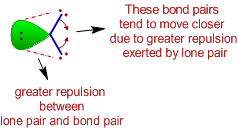ii) The repulsion between electron pairs increases with increase in electronegativity of central atom and hence the bond angle increases. The bond pairs are closer and thus by shortening the distance between them, which in turn increases the repulsion. Hence the bonds tend to move away from each other.The bond pairs tend to move away from each other since the distance between them is shortened as they are more localized on more electronegative central atom.

However the bond angle decreases when the electronegativities of ligand atoms are more than that of central atom. There is increase in the distance between bond pairs since they are now closer to ligand atoms. Due to this, they tend to move closer resulting in the decrease in bond angle.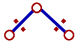The bond pairs tend to come closer since the distance between them is increased as they are more localized on more electronegative ligand atoms.

iii) The bond angle decreases with increase in the size of central atom.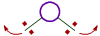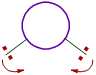On smaller central atoms the bond pairs are closer and hence tend to move away from each other so as to minimize repulsion. Hence bond angle will be more. On bigger central atoms, the bond pairs are more distant from each other and hence there is less repulsion. Hence they tend to move closer and thus by decreasing the bond angle.

However the bond angle increases with increase in the size of ligand atoms, which surround the central atom.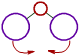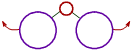There is less repulsion between smaller ligand atoms and they can move closer to each other and thus decrease the bond angle. These is more repulsion between bigger ligand atoms and hence they tend to move away from each other. Thus bond angle increases.

iv) The bond angles are also changed when multiple bonds are present. It is due to uneven repulsions.

6) When there are two or more resonance structures, the VSEPR theory is applicable to any of such contributing structure.

## RELATION BETWEEN NUMBER & TYPE OF VALENCE ELECTRON PAIRS WITH THE SHAPE OF MOLECULE

* The shape of molecule and also the approximate bond angles can be predicted from the number and type of electron pairs in the valence shell of central atom as tabulated below.

In the following table the molecule is represented by "AXE" notation, where

A = Central atom

X = Ligand atom bonded to the central atom either by a single bond or by multiple bond; indicating a bond pair.

E = Lone pair

Note:

* The sum of number of ligand atoms (X) and number of lone pairs (E) is also known as steric number.

* The bond pairs are shown as green colored  thick lines, whereas the lone pairs are shown as point charges using green colored lobes.

 Steric  number Number   of   Bond   pairs Number   of   Lone   pairs Formula Shape   of   molecule Approximate  Bond angles Examples 1 1 0 AX Linear- ClF, BrF, BrCl,  HF, O2 2 2 0 AX2 Linear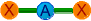180o BeCl2, HgCl2, CO2 3 3 0 AX3 Trigonal planar120o BF3, CO32-, NO3-,  SO3 2 1 AX2E Angular120o SO2, SnCl2, O3,  NSF, NO2- 4 4 0 AX4 Tetrahedral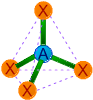109o28' CH4, SiCl4, NH4+,  PO43-, SO42-, ClO4- 3 1 AX3E Trigonal pyramidalaround 109o28' NH3, PCl3, XeO3 2 2 AX2E2 Angular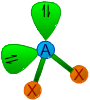around 109o28' H2O, SCl2, Cl2O, OF2 5 5 0 AX5 Trigonal bipyramidal120o & 90o PCl5, SOF4 4 1 AX4E See saw  or  distorted tetrahedral- SF4, TeCl4 3 2 AX3E2 T-Shape90o ClF3, BrF3, BrCl3 2 3 AX2E3 Linear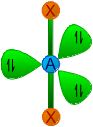180o XeF2, I3- 6 6 0 AX6 Octahedral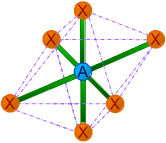90o SF6 5 1 AX5E Square pyramidal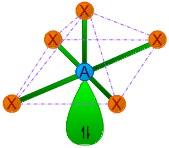90o ClF5, BrF5, ICl5 4 2 AX4E2 Square planar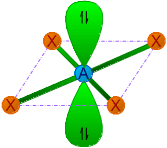90o XeF4 7 7 0 AX7 Pentagonal bipyramidal72o & 90o IF7 6 1 AX6E Pentagonal pyramidal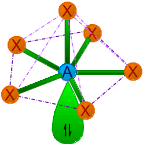around 72o & 90o XeOF5-, IOF52-

### STEPS INVOLVED IN PREDICTING THE SHAPES OF MOLECULES USING VSEPR THEORY

* The first step in determination of shape of a molecule is to write the Lewis dot structure of the molecule.

* Then find out the number of bond pairs and lone pairs in the valence shell of central atom.

While counting the number of bond pairs, treat multiple bonds as if they were single bonds. Thus electron pairs in multiple bonds are to be treated collectively as a single super pair.

* Use the above table to predict the shape of molecule based on steric number and the number of bond pairs and lone pairs.

## APPLICATIONS & ILLUSTRATIONS OF VSEPR THEORY

1) Methane (CH4):

* The Lewis structure of methane molecule is: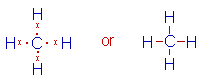* There are 4 bond pairs around the central carbon atom in its valence shell. Hence it has tetrahedral shape with 109o28' of bond angles.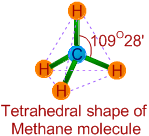2) Ammonia (NH3):

* The Lewis structure of ammonia indicates there are three bond pairs and one lone pair around the central nitrogen atom.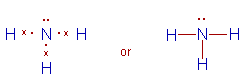* Since the steric number is 4, its structure is based on tetrahedral geometry. However, its shape is pyramidal with a lone pair on nitrogen atom.

* The bond angle is decreased from 109o28' to 107o48' due to repulsion caused by lone pair on the bond pairs.3) Water (H2O):

* It is evident from the Lewis structure of water molecule, there are two bond pairs and two lone pairs in the valence shell of oxygen. Hence its structure is based on tetrahedral geometry. However its shape is angular with two lone pairs on oxygen.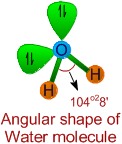* The bond angle is decreased to 104o28' due to repulsions caused by lone pairs on bond pairs. It can be noted that the bond angle decreases with increase in the number of lone pairs on the central atom.

4) Sulfur tetrachloride (SCl4):

Since there are four bond pairs and one lone pair around sulfur in its valence shell, the structure of SCl4 is based on trigonal bipyramidal geometry. It has seesaw shape with a lone pair occupying the equatorial position.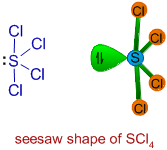The angles between P-Claxial and P-Clequatorial are less than 90o due to repulsion exerted by the lone pair. The angle between P-Clequatorial bonds also decreases from its usual value, 120o.

The lone pair occupies the equatorial position to minimize the repulsions.

Note: Usually the lone pairs, bulky groups and less electronegative atoms tend to occupy equatorial position to minimize repulsions. This is because they experience repulsion only from two groups at 90o, when they occupy the equatorial positions. However the repulsion will be more when they occupy axial positions, since they encounter three groups at 90o

5) PF3Cl2:

There are only 5 bond pairs on phosphorus atom. Hence it has trigonal bipyramidal shape. The chlorine atoms occupy the equatorial positions to minimize the repulsions since they are not only bulkier and also less electronegative than fluorine atoms.The bond pair of P-Cl is slightly more closer towards the P atom when compared to the bond pair of P-F, since the chlorine atoms are comparatively less electronegative than fluorine atoms. Hence there is comparatively more negative charge accumulation towards P atom, which makes the P-Cl bonds to experience more repulsion than P-F bonds. Hence they orient in equatorial positions at 120o to minimize repulsions.

Note that, here we are comparing the polarity of P-Cl bond with P-F bond. But one should keep in mind that the bond pair of P-Cl bond is still closer to Cl, since it is more electronegative than P atom.

6) Formaldehyde (HCHO):

There are three bond pairs around the central carbon atom. The double bond between C and O is considered as a single super pair. Hence the shape of the molecule is trigonal planar and the bond angles are expected to be equal to 120o

However, the C=O exerts more repulsion on the C-H bond pairs. Hence the ∠H-C-H bond angle will be less than 120o and the ∠H-C-O is greater than 120o.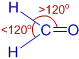Which of the following statements is false about VSEPR theory?

a) the geometry of a molecule is determined by the number of electron groups on the central atom.

b) the geometry of the electron groups is determined by minimizing repulsions between them.

c) a lone pair, a single bond, a double bond, a triple bond and a single electron - each of these is counted as a single electron group.

d) bond angles may depart from the idealized angles because lone pairs of electrons take up less space than bond pairs.

e) the number of electron groups can be determined from the Lewis structure of the molecule.

Hint:

Lone pairs are only attracted by one atom and bond pairs are attracted by two atoms.

 < Covalent bond : Lewis structures Chemical bonding: TOC Valence bond theory >